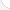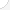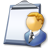Teachers-preschool, kindergarten, elementary, middle, and secondaryDescription: Teachers help students learn in school. They plan lessons, prepare tests, grade papers, and write reports of students' progress. They meet with parents and school staff to talk about grades and problems. Some teach a specific grade; others teach one subject to students in many grades. Most work more than 40 hours a week. Some work second jobs during the summer months. Many supervise school activities such as clubs and sports teams.Source: Bureau of Labor Statistics, U.S. Department of Labor, Occupational Outlook Handbook, 2008-09 Edition at http://www.bls.gov/OCO/Complete Job Profile: http://www.bls.gov/oco/ocos069.htmSalary: \$28,001 to \$50,000 per yearComments:I taught math in grades 6 through 12 for over 20 years. judyannb@verizon.net Judy Ann BrownI am a high school math teacher. I feel all topics listed are important to me, but the checked ones would be vital to all teachers. cdsflute@hotmail.com Cycelee HastingsMath is the blood in the veins of education. It is not possible to live in our world without the power of math. Math IS life! maryteach202@aol.com Mary LawrenceI use a lot more of these in my job as a math teacher (up through Pre-Calc), but I only included the ones that I USE as a teacher, not that I TEACH as a teacher. If I included those that I need to know to help my students understand their homework, I'd have checked almost everything in every category. KimOHara@aol.com Kim O'HaraI'm a Kindergarten teacher and I answered based on what I use in living my life as a teacher and a regular person, not what the children in my class use. I probably use more of the topics in First Year Algebra but since I don't really know what I'm talking about in Math, I don't really know what I use or not. I hope this was useful to you. Patricia

There are 71 math topics Teachers-preschool, kindergarten, elementary, middle, and secondary need to know.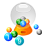Basic Math / Algebra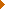FractionsDecimalsRatio and ProportionPercentCustomary MeasurementMetric MeasurementMeasurement ConversionBasic ProbabilityBasic StatisticsStatistical GraphingPowers and RootsOther Number BasesNegative NumbersScientific NotationBasic Problem Solving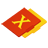First-Year AlgebraUsing FormulasLinear EquationsLinear InequalitiesOperations with PolynomialsFactoring PolynomialsRational ExpressionsCoordinate Graphing 2DLinear SystemsRadicalsQuadratic EquationsAlgebraic Representation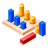GeometryBasic TerminologyAngle MeasurementCongruent TrianglesTriangle InequalitiesParallel LinesQuadrilateralsSimilarityGeometric MeanPythagorean TheoremRight Triangle TrigonometryCirclesConstructionsAreaVolumeTransformationsMake/Use 3D Drawings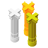Second-Year Algebra / TrigonometryFunctionsVariationImaginary NumbersPolynomial EquationsLogarithmsSequences and SeriesMatricesCoordinate Graphing 3DAdvanced ProbabilityAdvanced StatisticsConic SectionsNon-Linear SystemsTrigonometric/Circular FunctionsGraphs of Trigonometric FunctionsTrigonometric IdentitiesTrigonometric Equations/InversesOblique TrianglesPolar Coordinates/GraphsVectors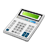Other TopicsCalculus and Higher MathBasic Calculator UseScientific Calculator UseComputer UseComputer ProgrammingGroup Problem SolvingMental MathInductive/Deductive ReasoningMath CommunicationsMathematical Modeling